# 深度学习入门（一），从Keras开始

### 文章目录####安装keras 安装环境：Anaconda(python3.6) 首先安装：tensorflow.（通过navigator,可参见[直通车](https://blog.csdn.net/hhtnan/article/details/79856084)） 检验：import tensorflow as tf Keras在anaconda下没有原装的安装包，只有使用pip安装方式,安装Keras，pip install Keras

import tensorflow as tf
import keras
print(tf.__version__)
print(keras.__version__)


Using TensorFlow backend.
1.2.1
2.1.6


**常见错误：**FutureWarning: Conversion of the second argument of issubdtype from float to np.floating is deprecated. In future, it will be treated as np.float64 == np.dtype(float).type.
from ._conv import register_converters as _register_converters
**解决方案：**pip install h5py==2.8.0rc1，安装 h5py，用于模型的保存和载入
**常见错误：**Using TensorFlow backend.

####1.关于Keras

1）简介

Keras是由纯python编写的基于theano/tensorflow的深度学习框架。Keras是一个高级神经网络API，用Python编写，能够在TensorFlow，CNTK或Theano之上运行。(官网：https://keras.io/)

Keras是一个高层神经网络API，支持快速实验，能够把你的idea迅速转换为结果，如果有如下需求，可以优先选择Keras：

a）简易和快速的原型设计（keras具有高度模块化，极简，和可扩充特性）
b）支持CNN和RNN，或二者的结合

c）无缝CPU和GPU切换

2）设计原则

a）用户友好：Keras是为人类而不是天顶星人设计的API。用户的使用体验始终是我们考虑的首要和中心内容。Keras遵循减少认知困难的最佳实践：Keras提供一致而简洁的API， 能够极大减少一般应用下用户的工作量，同时，Keras提供清晰和具有实践意义的bug反馈。

b）模块性：模型可理解为一个层的序列或数据的运算图，完全可配置的模块可以用最少的代价自由组合在一起。具体而言，网络层、损失函数、优化器、初始化策略、激活函数、正则化方法都是独立的模块，你可以使用它们来构建自己的模型。

c）易扩展性：添加新模块超级容易，只需要仿照现有的模块编写新的类或函数即可。创建新模块的便利性使得Keras更适合于先进的研究工作。

d）与Python协作：Keras没有单独的模型配置文件类型（作为对比，caffe有），模型由python代码描述，使其更紧凑和更易debug，并提供了扩展的便利性。
####2.Keras的模块结构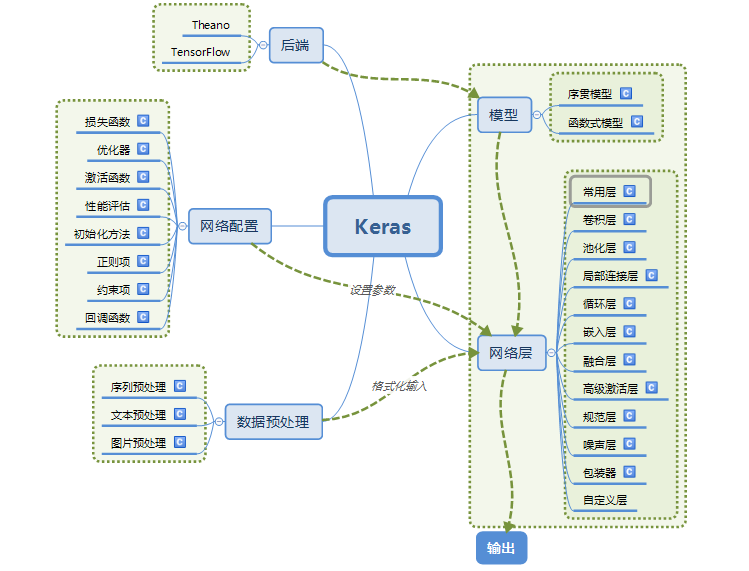####3.使用Keras搭建一个神经网络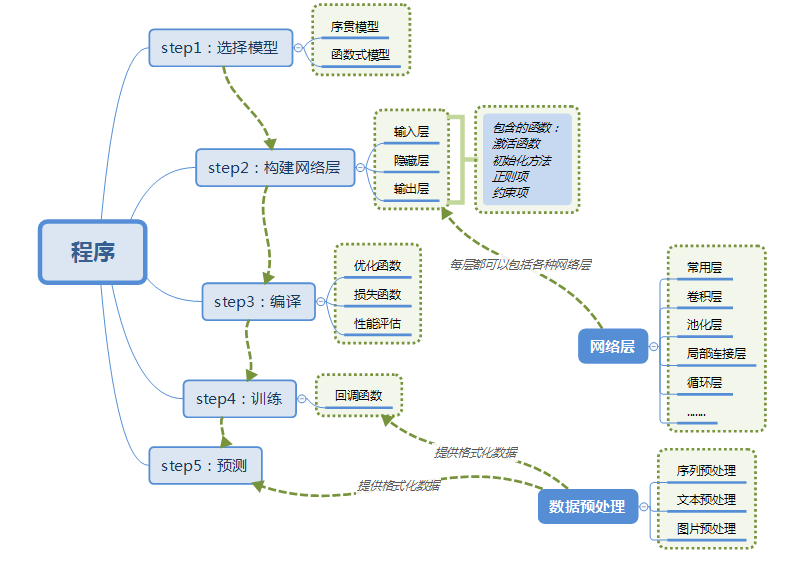####4.基本概念

1）符号计算

Keras的底层库使用Theano或TensorFlow，这两个库也称为Keras的后端。无论是Theano还是TensorFlow，都是一个“符号式”的库。符号计算首先定义各种变量，然后建立一个“计算图”,计算图规定了各个变量之间的计算关系。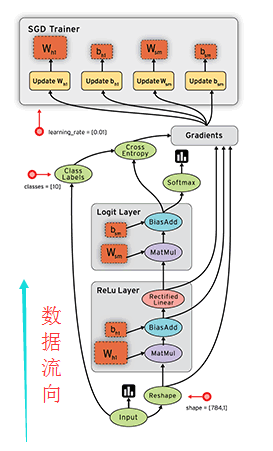2）数据格式(data_format)

    目前主要有两种方式来表示张量：
a) th模式或channels_first模式，Theano和caffe使用此模式。
b）tf模式或channels_last模式，TensorFlow使用此模式。

下面举例说明两种模式的区别：
对于100张RGB3通道的16×32（高为16宽为32）彩色图，
th表示方式：（100,3,16,32）
tf表示方式：（100,16,32,3）
唯一的区别就是表示通道个数3的位置不一样。


3）模型

      Keras有两种类型的模型，序贯模型（Sequential）和函数式模型（Model），函数式模型应用更为广泛，序贯模型是函数式模型的一种特殊情况。
a）序贯模型（Sequential):单输入单输出，一条路通到底，层与层之间只有相邻关系，没有跨层连接。这种模型编译速度快，操作也比较简单
b）函数式模型（Model）：多输入多输出，层与层之间任意连接。这种模型编译速度慢。


####5.第一个示例

      这里也采用介绍神经网络时常用的一个例子：手写数字的识别。

在写代码之前，基于这个例子介绍一些概念，方便大家理解。

PS：可能是版本差异的问题，官网中的参数和示例中的参数是不一样的，官网中给出的参数少，并且有些参数支持，有些不支持。所以此例子去掉了不支持的参数，并且只介绍本例中用到的参数。


1）Dense(500,input_shape=(784,))

a）Dense层属于网络层-->常用层中的一个层

b） 500表示输出的维度，完整的输出表示：(*,500)：即输出任意个500维的数据流。但是在参数中只写维度就可以了，比较具体输出多少个是有输入确定的。换个说法，Dense的输出其实是个N×500的矩阵。

c）input_shape(784,) 表示输入维度是784(28×28，后面具体介绍为什么)，完整的输入表示：(*,784)：即输入N个784维度的数据


2）Activation(‘tanh’)

a）Activation：激活层

b）‘tanh’ ：激活函数

3）Dropout(0.5)

4）数据集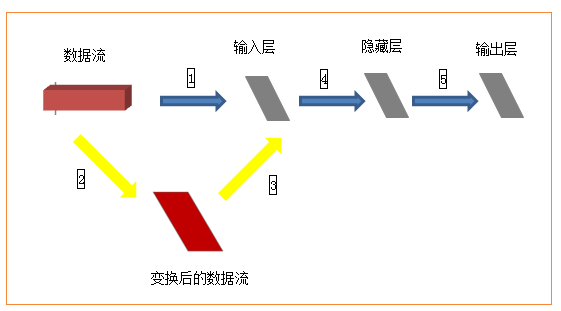####操作案例1

完整代码：
#1. 导入相关的Python和Keras的模块（module）
import numpy as np
np.random.seed(1337)
from keras.models import Sequential
from keras.layers import Dense

import matplotlib.pyplot as plt

# 1.Build the trainning data
X=np.linspace(-1,1,200)
np.random.shuffle(X)
np.random.normal()
Y=0.5*X+2+np.random.normal(0,0.05,(200,))
plt.scatter(X,Y)
plt.show()
X_train,Y_train=X[:160],Y[:160]
X_test,Y_test=X[160:],Y[160:]
print(X)
print("*******************************************")
print(Y)
# 2.Build a neural network from the 1st layer to the last layer
model=Sequential()

#3. Choose loss function and optimzing method
model.compile(loss='mse',optimizer='sgd')

#4. Trainning
print("Training......")
for step in range(1400):
cost=model.train_on_batch(X_train,Y_train)
if step % 100 ==0:
print('train cost',cost)

#5.Test
print("\n Testing...........")
cost=model.evaluate(X_test,Y_test,batch_size=40)
print("Test cost:",cost)
W,b=model.layers.get_weights()
print('Weight=',W,"\nbiases=",b)

#6.Plotting the prediction
Y_pred=model.predict(X_test)
plt.scatter(X_test,Y_test)
plt.plot(X_test,Y_pred)
plt.show()


Output: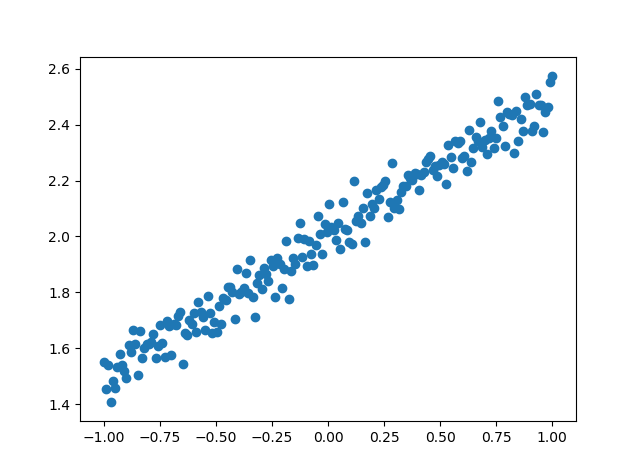train cost 4.0281153
train cost 0.080740646
train cost 0.0058854916
train cost 0.0031687208
train cost 0.002764651
train cost 0.0026669714
train cost 0.00264223
train cost 0.0026359423
train cost 0.0026343435
train cost 0.002633939
train cost 0.0026338336
train cost 0.002633807
train cost 0.002633801
train cost 0.0026337993

Testing...........

40/40 [==============================] - 0s 175us/step
Test cost: 0.0033766974229365587
Weight= [[0.5063296]]
biases= [2.0042796]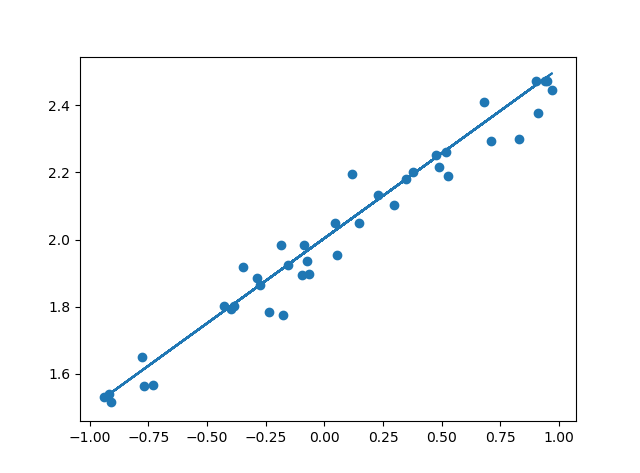#####案例1代码解读：

# 1.Build the trainning data
X=np.linspace(-1,1,200)
np.random.shuffle(X)
np.random.normal()
Y=0.5*X+2+np.random.normal(0,0.05,(200,))
plt.scatter(X,Y)
plt.show()
X_train,Y_train=X[:160],Y[:160]
X_test,Y_test=X[160:],Y[160:]
print(X)
print("*******************************************")
print(Y)


# 2.Build a neural network from the 1st layer to the last layer
model=Sequential()

#3. Choose loss function and optimzing method
model.compile(loss='mse',optimizer='sgd')


mean_squared_error
mean_absolute_error
mean_absolute_percentage_error
mean_squared_logarithmic_error
squared_hinge
hinge
logcosh
categorical_crossentropy
sparse_categorical_crossentropy
binary_crossentropy
kullback_leibler_divergence
poisson
cosine_proximity


https://github.com/fchollet/keras/blob/master/keras/losses.py

RMSprop
TFOptimizer


#4. Trainning
print("Training......")
for step in range(1400):
cost=model.train_on_batch(X_train,Y_train)
if step % 100 ==0:
print('train cost',cost)


#5.Test
print("\n Testing...........")
cost=model.evaluate(X_test,Y_test,batch_size=40)
print("Test cost:",cost)
W,b=model.layers.get_weights()
print('Weight=',W,"\nbiases=",b)


#6.Plotting the prediction
Y_pred=model.predict(X_test)
plt.scatter(X_test,Y_test)
plt.plot(X_test,Y_pred)
plt.show()


Keras模型结果 VS 原始测试数据结果

####案例2

"""
from keras.models import Sequential
from keras.layers.core import Dense, Dropout, Activation
from keras.optimizers import SGD
from keras.datasets import mnist
import numpy
'''
第一步：选择模型
'''
model = Sequential()
'''
第二步：构建网络层
'''

'''
第三步：编译
'''
sgd = SGD(lr=0.01, decay=1e-6, momentum=0.9, nesterov=True) # 优化函数，设定学习率（lr）等参数
model.compile(loss='categorical_crossentropy', optimizer=sgd, class_mode='categorical') # 使用交叉熵作为loss函数

'''
第四步：训练
.fit的一些参数
batch_size：对总的样本数进行分组，每组包含的样本数量
epochs ：训练次数
shuffle：是否把数据随机打乱之后再进行训练
validation_split：拿出百分之多少用来做交叉验证
verbose：屏显模式 0：不输出  1：输出进度  2：输出每次的训练结果
'''
(X_train, y_train), (X_test, y_test) = mnist.load_data() # 使用Keras自带的mnist工具读取数据（第一次需要联网）
# 由于mist的输入数据维度是(num, 28, 28)，这里需要把后面的维度直接拼起来变成784维
X_train = X_train.reshape(X_train.shape, X_train.shape * X_train.shape)
X_test = X_test.reshape(X_test.shape, X_test.shape * X_test.shape)
Y_train = (numpy.arange(10) == y_train[:, None]).astype(int)
Y_test = (numpy.arange(10) == y_test[:, None]).astype(int)

model.fit(X_train,Y_train,batch_size=200,epochs=50,shuffle=True,verbose=0,validation_split=0.3)
model.evaluate(X_test, Y_test, batch_size=200, verbose=0)

'''
第五步：输出
'''
print("test set")
scores = model.evaluate(X_test,Y_test,batch_size=200,verbose=0)
print("")
print("The test loss is %f" % scores)
result = model.predict(X_test,batch_size=200,verbose=0)

result_max = numpy.argmax(result, axis = 1)
test_max = numpy.argmax(Y_test, axis = 1)

result_bool = numpy.equal(result_max, test_max)
true_num = numpy.sum(result_bool)
print("")
print("The accuracy of the model is %f" % (true_num/len(result_bool)))


https://blog.csdn.net/zhzhx1204/article/details/77393245

11-26
04-02247901-111万+
01-04333
08-1712万+
05-017802
05-236万+
10-192万+
11-102万+
04-048184点击重新获取扫码支付余额充值